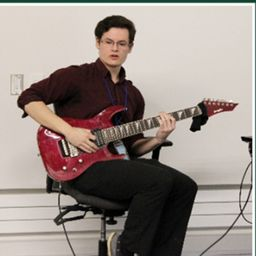🚨 Hurry, space in our FREE summer bootcamps is running out. 🚨Claim your spot here.# Lucas F.

Carleton University

## Biography

I struggled with Math a lot when I was young, until I began to see the beauty of the topic, thanks to a Metal band of all things. I googled "Tesseract" one day, and found the wikipedia page for the Four-Dimensional analogue to the cube. That absolutely blew my mind. "Math can describe things that human beings can't even imagine?" The next step was seeing the film "Interstellar", and seeing the blackboards full of equations. That made me want to study math and physics until I could understand those equations.

Now, I'm pursuing my Master's degree, doing research on resonance phenomena in epidemiological equations. I've taught tutorials in Linear Algebra, Complex and Real Analysis, and Calculus, in addition to being a private tutor working with high school students as well as university students.

## Education

MS Applied Mathematics
Carleton University
BS Mathematical Physics
Trent University

## Topics Covered

Quantum Physics
Atomic Physics
Functions of Several Variables
Limits
Continuous Functions
Vector Calculus
Probability Topics
Trigonometry
An Introduction to Geometry
Differentiation
Integrals
Applications of Integration
Temperature and Heat
Partial Derivatives
Matrices
Systems of Equations and Inequalities
Introduction to Matrices
Functions
Polynomials
Rational Functions
Graphs and Statistics
Equations and Inequalities
Derivatives
Applications of the Derivative
Vectors
Vector Functions
Linear Functions
Parametric Equations
Polar Coordinates
Complex Numbers
Integration
Integration Techniques
Introduction to Conic Section
Functions
Algebra Topics That are Reviewed at the Start of the Semester
Introduction to Trigonometry
Series
1 2 3 4 5 ... 79